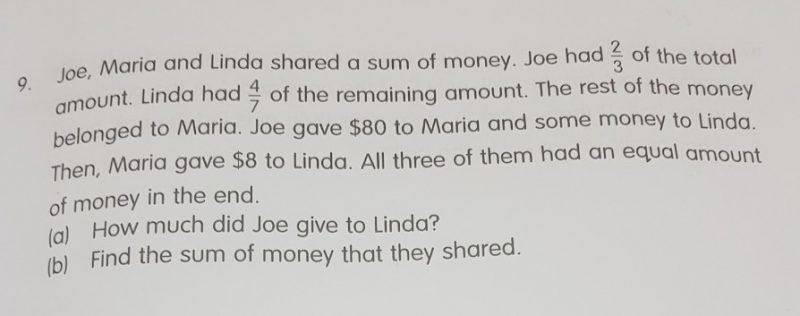QuestionAssuming money that:

Linda has 4 unit, (4/7 of remaining)

Maria has 3 unit (3/7 of remaining)

Joe has 14 unit ((4+3) x 2 unit)

Total 21 unit of money, if share equally, each will ha 7 unit.

For Maria,

3 unit + \$80 – \$8 = 7 unit

4 unit = \$72

1 unit = \$18

Amount Joe gave to Linda will be,

(\$18 x 7) – \$80 = \$46

a) Joe gave \$46 to Linda.

\$18 x 21 = \$378

b) Total amount of money will be \$378